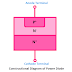A Power Diode is basically a normal PN Junction Diode used in Power Electronic circuits for the operation of high current and high power applications, that is why it is called Power Diode. It can be a definition of a power diode. Power Diode also has two terminals (Anode and Cathode) like a conventional PN Junction Diode but there is a difference between the construction of a Power Diode and a normal diode. It is a special type of diode. In this article, we are going to know the construction, working principle, types, and applications of a power diode.

## Power Diode Construction

Here, you can see the constructional diagram of a power diode.

Power diode is also a two-terminal, two-layer, and single-junction device. It has a P-layer and N-layer. Also, you can see the N Layer is divided into two sub layer N+ and N-. In normal diodes, there are two-layer P+ and N+, but in the case of a power diode, an additional sub-layer is available that is N- layer. This N- layer helps to use the power diode for high power applications.

Here, the P+ layer is heavily doped, the N+ layer is heavily doped, but the N- Layer is very lightly doped. It is almost intrinsic in nature. Power Diode is also known as PIN Diode due to the existence of the N- layer with intrinsic nature. Here, I indicate the term Intrinsic.

The thickness of the depletion region or space charge region of a power diode is larger than the normal diode. This helps to block a high reverse current without breaking down. This feature also makes a power diode very efficient.

## Power Diode V-I Characteristics

Here, you can see the V-I Characteristics diagram of a Power Diode.

The characteristics of a power diode are very similar to a normal signal diode. In the forward bias condition, the current flow through the diode increase and decrease linearly with the increasing and decreasing of applied voltage. As the power diode conducts a very high current, so the ohmic drop is also very high and it makes an exponential growth.

## Working Principle of Power Diode

When a forward voltage is applied across the power diode or the voltage of the anode terminal is higher than the cathode terminal, the power diode conducts a forward current through it. If the applied voltage is increased then the flow of current also be increased. Power diodes have very low forward voltage drop characteristics due to their advanced design. Generally, the forward voltage drop in a power diode exists between 0.5V to 1.2V.

When a reverse voltage is applied across the diode or the voltage of the cathode terminal is higher than the anode terminal, it does not allow the flow of current through it. In this mode, it blocks the current.

## Power Diode Types

There are different types of power diodes available based on the reverse recovery time and manufacturing design, such as,
• General Power Diode
• Fast Recovery
• Schottky Diode

## Use and Applications of Power Diode

1. Power Diodes are used in high power applications such as three-phase rectifiers, inverters.
2. Power diodes are used for power controllers, battery chargers, control panel power supplies, etc.
3. Power diodes are used in snubber circuits, high voltage rectifications, etc.

1. Power diode Capable to handle a large amount of current for a long time.

2. It can block a very high current in reverse bias.

3. It provides a very high efficiency than normal diodes.

1. Power Diodes are costlier than normal diodes.

2. Power diode generates more heat than a normal diode, so always a heat sink has to use with power diodes.

3. Power Diode makes more power loss than normal diodes.

What is Power Diode? Applications, Use, Working Principle, TypesReviewed by Author on October 29, 2021 Rating: 5